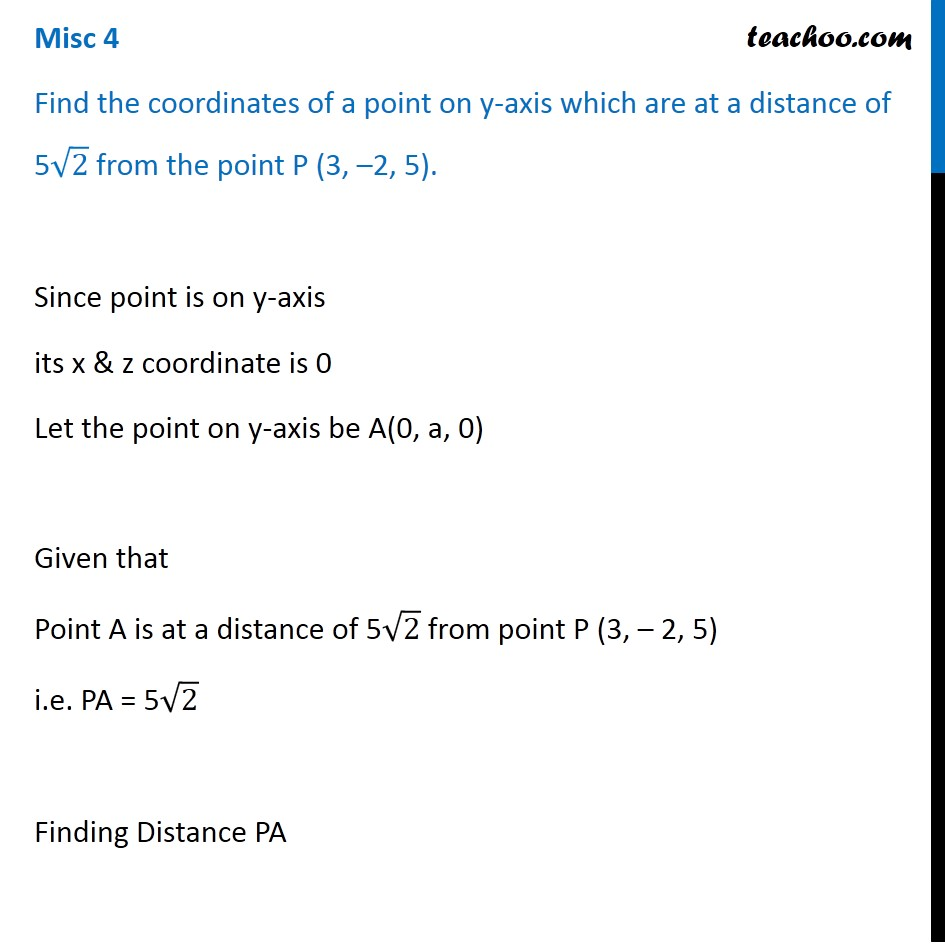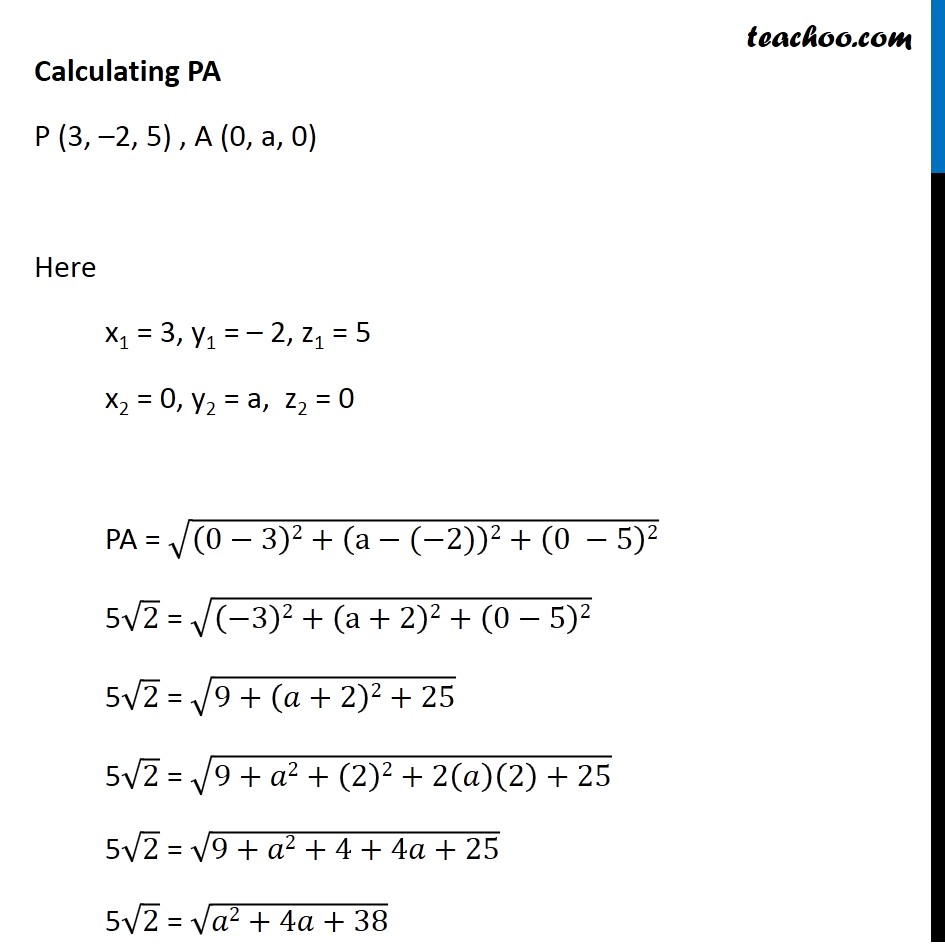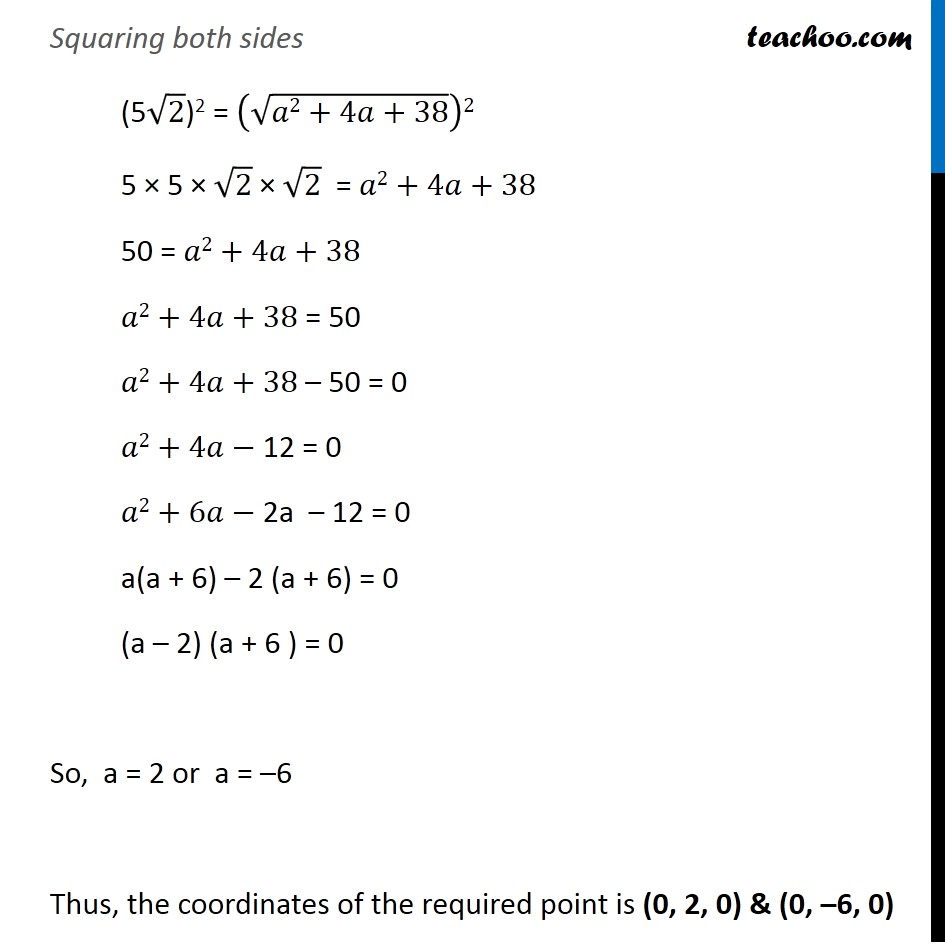Miscellaneous

Chapter 11 Class 11 - Intro to Three Dimensional Geometry
Serial order wiseLearn in your speed, with individual attention - Teachoo Maths 1-on-1 Class

### Transcript

Misc 4 Find the coordinates of a point on y-axis which are at a distance of 5√2 from the point P (3, –2, 5). Since point is on y-axis its x & z coordinate is 0 Let the point on y-axis be A(0, a, 0) Given that Point A is at a distance of 5√2 from point P (3, – 2, 5) i.e. PA = 5√2 Finding Distance PA Calculating PA P (3, –2, 5) , A (0, a, 0) Here x1 = 3, y1 = – 2, z1 = 5 x2 = 0, y2 = a, z2 = 0 PA = √((0−3)2+(a−(−2))2+(0 −5)2) 5√2 = √((−3)2+(a+2)2+(0−5)2) 5√2 = √(9+(𝑎+2)2+25) 5√2 = √(9+𝑎2+(2)2+2(𝑎)(2)+25) 5√2 = √(9+𝑎2+4+4𝑎+25) 5√2 = √(𝑎2+4𝑎+38) Squaring both sides (5√2)2 = (√(𝑎2+4𝑎+38))2 5 × 5 × √2 × √2 = 𝑎2+4𝑎+38 50 = 𝑎2+4𝑎+38 𝑎2+4𝑎+38 = 50 𝑎2+4𝑎+38 – 50 = 0 𝑎2+4𝑎− 12 = 0 𝑎2+6𝑎− 2a – 12 = 0 a(a + 6) – 2 (a + 6) = 0 (a – 2) (a + 6 ) = 0 So, a = 2 or a = –6 Thus, the coordinates of the required point is (0, 2, 0) & (0, –6, 0)